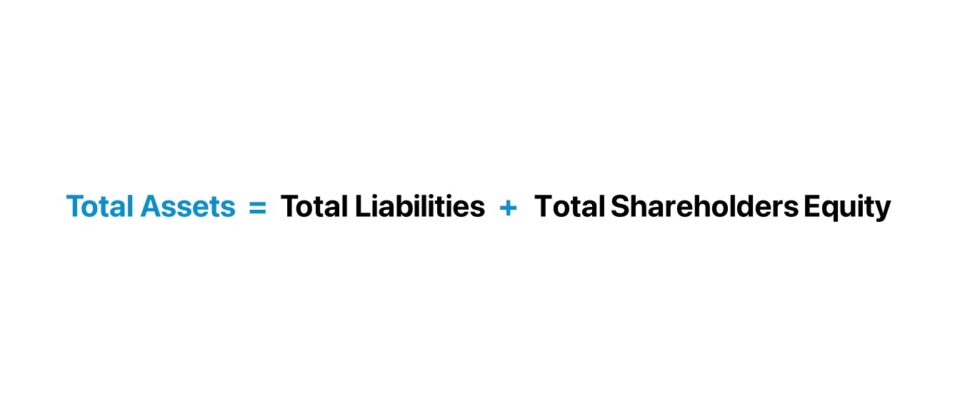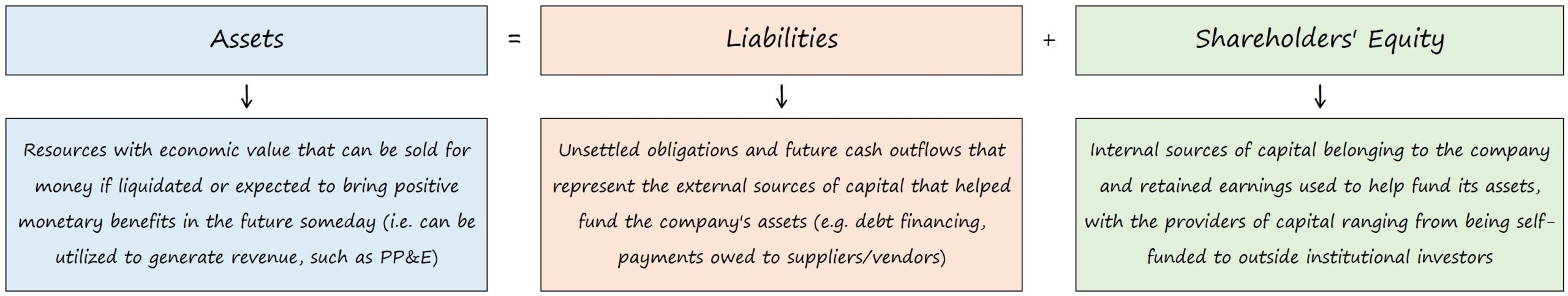Welcome to Wall Street Prep! Use code at checkout for 15% off.# Accounting Equation

Guide to Understanding the Fundamental Accounting Equation (Assets = Liabilities + Equity)## Fundamental Accounting Equation (Assets = Liabilities + Equity)

The accounting equation states that a company’s assets must be equal to the sum of its liabilities and equity, at all times.

The accounting equation is a core principle in the double-entry bookkeeping system, wherein each transaction must affect at a bare minimum two of the three accounts, i.e. a debit and credit entry.

There are three components to the accounting equation:

1. Assets → An asset is a resource owned by a company with positive economic value, which either possesses monetary value (i.e. can be sold for a net gain) or is expected to produce future benefits, such as PP&E. Assets can either be tangible, like fixed assets (e.g. equipment and machinery) or intangible (e.g. patents, trademarks, intellectual property and copyrights).
2. Liabilities → A liability represents a company’s obligations that represent future outflows of cash, i.e. unmet payments. For instance, common liabilities include debt securities such as loans, accounts payable (A/P), accrued expenses and deferred revenue.
3. Equity → The equity section of the balance sheet, often called “Shareholders’ Equity”, is the net assets of a company, i.e. the remaining value of a company’s assets after subtracting liabilities. The equity component is composed of items such as paid-in capital, retained earnings and treasury stock.## What are the Components of the Accounting Equation?

The balance sheet is one of the three main financial statements that depicts a company’s assets, liabilities, and equity sections at a specific point in time (i.e. a “snapshot”).

Typically reported quarterly or annually, the balance sheet consists of three components:

Balance Sheet Description
Assets Section
• The resources with economic value, which can be sold for money post-liquidation or are expected to bring positive monetary benefits in the future.
Liabilities Section
• The unsettled future obligations to a third party that represent economic costs (i.e. the external sources of capital from third parties that helped fund the company’s purchase of assets).
Shareholders’ Equity Section
• The internal sources of capital that helped fund its assets, such as capital invested by the founders and issuances of equity financing.

## Accounting Equation Formula

The fundamental accounting equation, as mentioned earlier, is as follows:

Total Assets = Total Liabilities + Total Shareholders’ Equity

The rationale is that the assets belonging to a company must have been funded somehow, i.e. the money used to purchase the assets did not just appear out of thin air to state the obvious.

If a company’s assets were hypothetically liquidated (i.e. the difference between assets and liabilities), the remaining value is the shareholders’ equity account.

Therefore, the assets side must always be equal to the sum of the liabilities and equity — which are the company’s two funding sources:

1. Liabilities — e.g. Accounts Payable, Accrued Expenses, Debt Financing
2. Shareholders’ Equity — e.g. Common Stock and APIC, Retained Earnings

## What is the Double Entry Accounting System?

The accounting equation sets the foundation of “double-entry” accounting, since it shows a company’s asset purchases and how they were financed (i.e. the off-setting entries).

A company’s “uses” of capital (i.e. the purchase of its assets) should be equivalent to its “sources” of capital (i.e. debt, equity).

In all financial statements, the balance sheet should always remain in balance.

Under the double-entry accounting system, each recorded financial transaction results in adjustments to a minimum of two different accounts.

On the accounting ledger, there are two entries recorded for bookkeeping purposes:

1. Debits — An entry on the left side of the ledger
2. Credits — An entry on the right side of the ledger

Each entry on the debit side must have a corresponding entry on the credit side (and vice versa), which ensures the accounting equation remains true.

For all recorded transactions, if the total debits and credits for a transaction are equal, then the result is that the company’s assets are equal to the sum of its liabilities and equity.Step-by-Step Online Course

### Everything You Need To Master Financial Modeling

Enroll in The Premium Package: Learn Financial Statement Modeling, DCF, M&A, LBO and Comps. The same training program used at top investment banks.

Inline Feedbacks